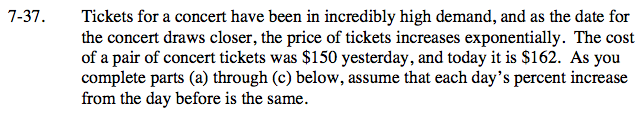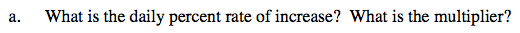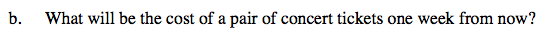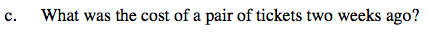### Home > CCA > Chapter 7 > Lesson 7.1.3 > Problem7-37

7-37.
1. Tickets for a concert have been in incredibly high demand, and as the date for the concert draws closer, the price of tickets increases exponentially. The cost of a pair of concert tickets was $150 yesterday, and today it is$162. As you complete parts (a) through (c) below, assume that each day’s percent increase from the day before is the same. Homework Help ✎

1. What is the daily percent rate of increase? What is the multiplier?

2. What will be the cost of a pair of concert tickets one week from now?

3. What was the cost of a pair of tickets two weeks ago?What percent of $150 is$162?Construct a table of values or an equation describing the relation between the number of days since yesterday and the price of a ticket.In order to go backwards, make the exponent negative.